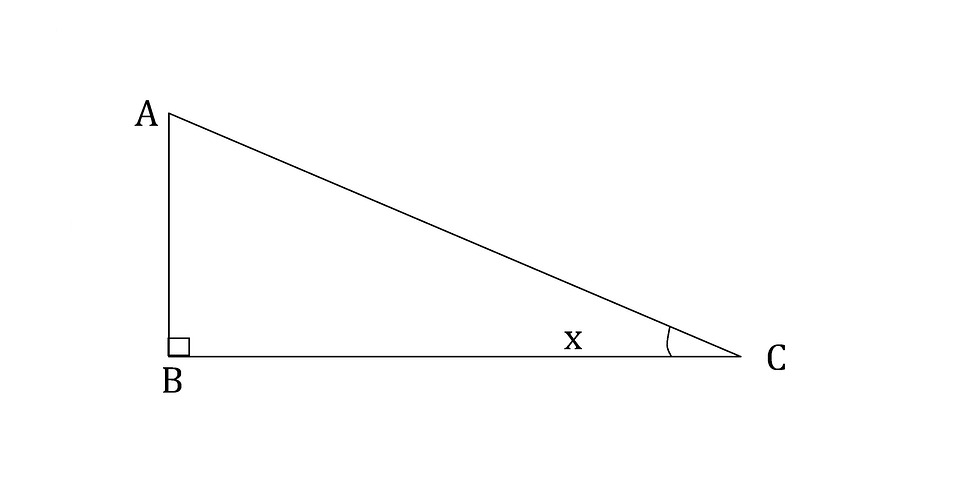# Trigonometric Identities

1) $sin^2$ x + $cos^2$ x = 1

proof:

To prove this identity let us use pythagoras theorem. consider a right angled triangle as shown in the figure. It is a triangle ABC with right angle at the vertex B and angle x at vertex C.

According to pythagoras theorem we can write$AB^2$ + $BC^2$ = $AC^2$

Divide with $AC^2$ on both sides.

$\frac{AB^2+BC^2}{AC^2}$ = 1

$\frac{AB^2}{AC^2}$ + $\frac{BC^2}{AC^2}$ = 1

by definition sin x = $\frac{opposite\ side\ to \ angle\ x}{hypotenuse}$ = $\frac{AB}{AC}$ and cos x = $\frac{adjacent \ side \ to\ angle \ x}{hypotenuse}$ = $\frac{CB}{AC}$

Therefore $sin^2$ x + $cos^2$ x = 1

I) Practice problems

1. If sin x = $\frac{4}{5}$ find the value of cos x provided x is acute angle.
2. find the value of $sin^2$ $50^0$ + $sin^2$ $40^0$
3. find th value of $sin^2$ $18^0$ + $sin^2$ $72^0$ + $sin^2$ $108^0$ + $sin^2$ $162^0$

Solutions at the end of the article.

2) $sec^2$ x - $tan^2$ x =1

proof:

To prove this identity let us use pythagoras theorem. consider a right angled triangle as shown in the figure. It is a triangle ABC with right angle at the vertex B and angle x at vertex C.According to pythagoras theorem we can write

$AB^2$ + $BC^2$ = $AC^2$

Divide with $BC^2$ on both sides, so that we can get tan x and sec x functions in the equation.

$\frac{AB^2}{BC^2}$ + 1 = $\frac{AC^2}{BC^2}$

by definition tan x = $\frac{opposite\ side\ to\ angle\ x}{adjacent\ side\ to\ angle\ x}$ = $\frac{AB}{BC}$ and sec x = $\frac{hypotenuse}{adjacent\ side\ to\ angle\ x}$ = $\frac{AC}{BC}$

Therefore $tan^2$ x + 1 = $sec^2$ x

II) solve the following problem

1. If sec A + tan A = 4, find the value of sin A.
2. Prove that $\frac{tan\ A+sec\ A -1}{tan\ A-sec\ A +1}$ = $\frac{1+sin\ A}{cos\ A}$

Solutions at the end of the article.

3) $cosec^2$ x - $cot^2$ x =1

proof:

To prove this identity let us use pythagoras theorem. consider a right angled triangle as shown in the figure. It is a triangle ABC with right angle at the vertex B and angle x at vertex C.According to pythagoras theorem we can write

$AB^2$ + $BC^2$ = $AC^2$

Divide with $AB^2$ on both sides, so that we can get tan x and sec x functions in the equation.

$\frac{BC^2}{AB^2}$ + 1 = $\frac{BC^2}{AC^2}$

by definition cot x = $\frac{adjacent\ side\ to\ angle\ x}{opposite\ side\ to\ angle\ x}$ = $\frac{BC}{AB}$ and cosec x = $\frac{hypotenuse}{opposite\ side\ to\ angle\ x}$ = $\frac{BC}{AC}$

Therefore $cosec^2$ x - $cot^2$ x =1

III) solve the following

1. find the value of (sin x + cosec x) $^2$ + (cos x + sex x) $^2$ - (tan x + cot x) $^2$
2. If Cosex A + Cot A = $\frac{2}{3}$ find the value of Sin A

Solutions at the end of the article.

Solutions

I)

1. ans= $\frac{3}{5}$

sol: Given Sin x = $\frac{4}{5}$

using the identity of trigonometry

$sin^2$ x + $cos^2$ x = 1

$\frac{4^2}{5^2}$ + $cos^2$ x = 1

$cos^2$ x = $\frac{9}{25}$

cos x = + $\frac{3}{5}$ or - $\frac{3}{5}$

1. ans= 1

sol:

$sin^2$ $50^0$ + $sin^2$ $40^0$

= $sin^2$ $50^0$ + $sin^2$ $(90^0-50^0)$

= $sin^2$ $50^0$ + $cos^2$ $50^0$

use the identity of trigonometry $sin^2$ x + $cos^2$ x = 1

= 1

1. ans= 2

sol:

$sin^2$ $18^0$ + $sin^2$ $72^0$ + $sin^2$ $108^0$ + $sin^2$ $162^0$

= $sin^2$ $18^0$ + $sin^2$ $72^0$ + $sin^2$ $(90^0+18^0)$ + $sin^2$ $(90^0+72^0)$

= $sin^2$ $18^0$ + $sin^2$ $72^0$ + $cos^2$ $18^0$ + $cos^2$ $72^0$

= ($sin^2$ $18^0$ + $cos^2$ $18^0$ ) + ( $sin^2$ $72^0$ + $cos^2$ $72^0$ )

use the identity of trigonometry $sin^2$ x + $cos^2$ x = 1

= 2

II)

1. Ans= $\frac{15}{17}$

Sol:

given sec A+tan A=4

let’s use the trigonometric identity

$sec^2$ x - $tan^2$ x =1

use the algebraic equation $a^2-b^2$=(a+b)(a-b)

(sec A + tan A)(Sec A - tan A) =1

4*(Sec A - tan A) =1

Sec A - tan A = $\frac{1}{4}$

(Sec A + tan A)+(Sec A - tan A)= 4+ $\frac{1}{4}$

sec A = $\frac{17}{8}$

similarly subtracting the two equations

(Sec A + tan A)-(Sec A - tan A) = 4- $\frac{1}{4}$

tan A = $\frac{15}{8}$

on divinding tan A with sec A we get sin A

sin A = $\frac{15}{8}$ * $\frac{8}{17}$

sin A = $\frac{15}{17}$

Sol:

Consider LHS

$\frac{tan\ A+sec\ A -1}{tan\ A-sec\ A +1}$

multiply and divide the fraction with $tan\ A+sec\ A +1$

= $\frac{ [ (tan\ A+sec\ A) -1 ][(tan\ A+sec\ A) +1 ]}{[(tan\ A+1)-sec\ A][(tan\ A+1) +sec\ A]}$

= $\frac{ (tan\ A+sec\ A)^2 -1}{(tan\ A+1)^2-sec^2\ A}$

= $\frac{tan^2\ A+ sec^2\ A+2tan\ A sec\ A -1}{tan^2\ A+2tan\ A+1-sec^2\ A}$

use the trigonometric identity $sec^2$ x - $tan^2$ x =1 which can also be written as $sec^2$ x -1= $tan^2$ x

= $\frac{2tan^2\ A +2tan\ A sec\ A}{2tan\ A+1-1}$

= tan A + sec A

= $\frac{sin\ A}{cos\ A}$ + $\frac{1}{cos\ A}$

= $\frac{1+sin\ A}{cos\ A}$

III)

1. ans = 5 Sol:

(sin x + cosec x) $^2$ + (cos x + sex x) $^2$ - (tan x + cot x) $^2$

= $sin^2$ x + $cosec^2$ x + 2+ $cos^2$ x+ $sec^2$ x+ 2 - $tan^2$ x - $cot^2$ x-2

= ( $sin^2$ x + $cos^2$ x)+( $cosec^2$ x- $cot^2$ x) + ( $sec^2$ x- $tan^2$ x) + 2

= 5

1. ans= $\frac{3}{13}$

Sol:

Cosex A + Cot A = $\frac{2}{3}$

let’s use the trigonometric identity

$cosec^2$ x - $cot^2$ x =1

use the algebraic equation $a^2-b^2$=(a+b)(a-b)

(Cosex A + Cot A)(Cosex A - Cot A)=1

$\frac{2}{3}$ (Cosex A - Cot A)=1

(Cosex A - Cot A)= $\frac{3}{2}$

(Cosex A + Cot A)+(Cosex A - Cot A)= $\frac{2}{3}$ + $\frac{3}{2}$
cosec A = $\frac{13}{3}$
sin A = $\frac{3}{13}$